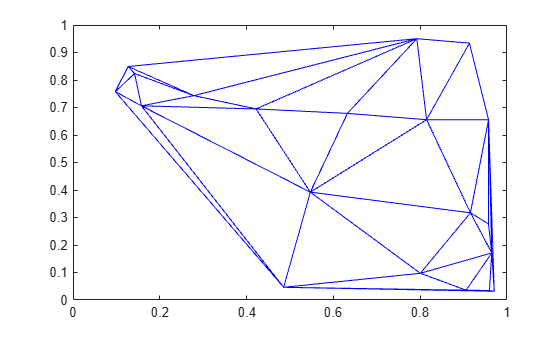# delaunay

Delaunay triangulation

## Syntax

``DT = delaunay(P)``
``DT = delaunay(x,y)``
``DT = delaunay(x,y,z)``

## Description

````DT = delaunay(P)` creates a 2-D or 3-D Delaunay triangulation from the points in a matrix `P`. The output `DT` is a three-column (for two dimensions) or four-column (for three dimensions) matrix where each row contains the row indices of the input points that make up a triangle or tetrahedron in the triangulation.```

example

````DT = delaunay(x,y)` creates a 2-D Delaunay triangulation from the points in vectors `x` and `y`.```
````DT = delaunay(x,y,z)` creates a 3-D Delaunay triangulation from the points in vectors `x`, `y`, and `z`.```

## Examples

collapse all

Create a set of 2-D points. Compute and plot the Delaunay triangulation.

```rng default; x = rand([20,1]); y = rand([20,1]); DT = delaunay(x,y); triplot(DT,x,y);```## Input Arguments

collapse all

Points, specified as a matrix whose columns are the x-coordinates, y-coordinates, and (possibly) z-coordinates of the triangulation points. Each row of the output `DT` contains the row numbers of `P` that make up a single triangle or tetrahedron in the triangulation.

x-coordinates, specified as a column vector.

y-coordinates, specified as a column vector.

z-coordinates, specified as a column vector.